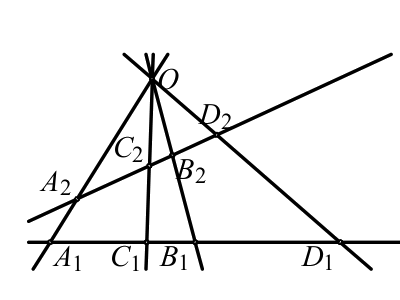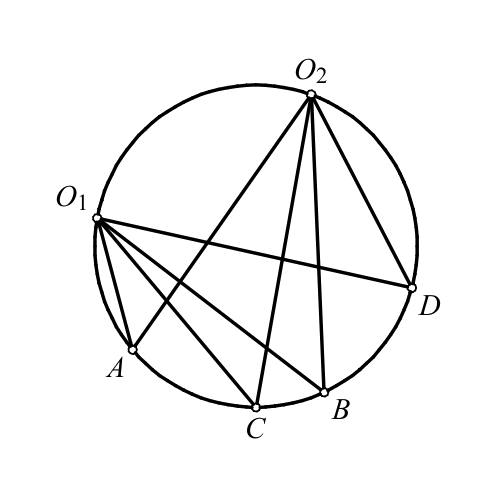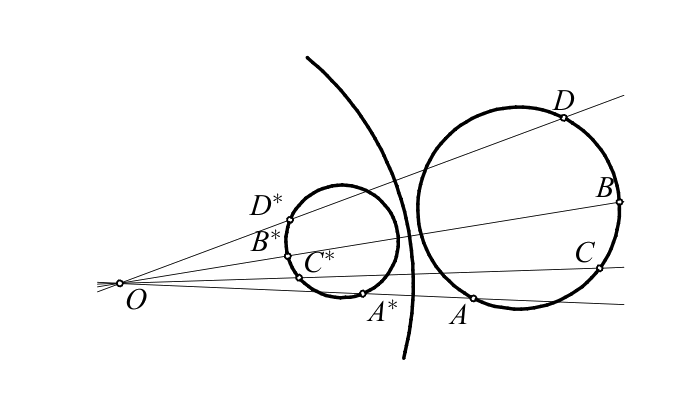## Cross ratio. Harmonic conjugates. Perspectivity. Projectivity

Definition Let $$A$$, $$B$$, $$C$$, and $$D$$ be colinear points. The cross ratio of the pairs of points $$(A,B)$$ and $$(C,D)$$ is $\mathcal{R}(A,B;C,D) = \frac{\overrightarrow{AC}} {\overrightarrow{CB}}:\frac{\overrightarrow{AD}} {\overrightarrow{DB}}. \quad\quad\quad\quad\quad (1)$

Let $$a$$, $$b$$, $$c$$, $$d$$ be four concurrent lines. For the given lines $$p_1$$ and $$p_2$$ let us denote $$A_i=a\cap p_i$$, $$B_i=b\cap p_i$$, $$C_i=c\cap p_i$$, $$D_i=d\cap p_i$$, for $$i=1$$, $$2$$. Then $\mathcal R(A_1,B_1;C_1,D_1)= \mathcal R(A_2,B_2;C_2,D_2).\quad\quad\quad\quad\quad (2)$Thus it is meaningful to define the cross ratio of the pairs of concurrent lines as $\mathcal R(a,b;c,d)=\mathcal R(A_1,B_1;C_1,D_1). \quad\quad\quad\quad\quad (3)$Assume that points $$O_1$$, $$O_2$$, $$A$$, $$B$$, $$C$$, $$D$$ belong to a circle. Then $\mathcal R(O_1A,O_1B;O_1C,O_1D) = \mathcal R(O_2A,O_2B;O_2C,O_2D).\quad\quad\quad\quad\quad (4)$ Hence it is meaningful to define the cross-ratio for cocyclic points as $\mathcal R(A,B;C,D) =\mathcal R(O_1A,O_1B;O_1C,O_1D).\quad\quad\quad\quad\quad (5)$ Assume that the points $$A$$, $$B$$, $$C$$, $$D$$ are colinear or cocyclic. Let an inversion with center $$O$$ maps $$A$$, $$B$$, $$C$$, $$D$$ into $$A^*$$, $$B^*$$, $$C^*$$, $$D^*$$. Then $\mathcal R(A,B;C,D)=\mathcal R(A^*,B^*;C^*,D^*). \quad\quad\quad\quad\quad (6)$Definition Assume that $$A$$, $$B$$, $$C$$, and $$D$$ are cocyclic or colinear points. Pairs of points $$(A,B)$$ and $$(C,D)$$ are harmonic conjugates if $$\mathcal R(A,B;C,D)=-1$$. We also write $$\mathcal H(A,B;C,D)$$ when we want to say that $$(A,B)$$ and $$(C,D)$$ are harmonic conjugates to each other.

Definition Let each of $$l_1$$ and $$l_2$$ be either line or circle. Perspectivity with respect to the point $$S$$ (denote as $$\frac{S}{\overline\wedge}$$), is the mapping of $$l_1\rightarrow l_2$$, such that

(i) If either $$l_1$$ or $$l_2$$ is a circle than it contains $$S$$;

(ii) every point $$A_1\in l_1$$ is mapped to the point $$A_2=OA_1\cap l_2$$.

According to the previous statements perspectivity preserves the cross ratio and hence the harmonic conjugates.

Definition Let each of $$l_1$$ and $$l_2$$ be either line or circle. Projectivity is any mapping from $$l_1$$ to $$l_2$$ that can be represented as a finite composition of perspectivities.

Theorem 1 Assume that the points $$A$$, $$B$$, $$C$$, $$D_1$$, and $$D_2$$ are either colinear or cocyclic. If the equation $$\mathcal R(A,B;C,D_1)= \mathcal R(A,B;C,D_2)$$ is satisfied, then $$D_1=D_2$$. In other words, a projectivity with three fixed points is the identity.

Theorem 2 If the points $$A$$, $$B$$, $$C$$, $$D$$ are mutually discjoint and $$\mathcal R(A,B;C,D)=\mathcal R(B,A;C,D)$$ then $$\mathcal H(A,B;C,D)$$.